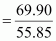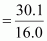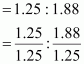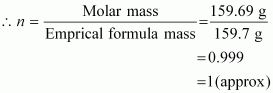# Determine the molecular formula of an oxide of iron in which the mass per cent

9 years ago

Mass percent of iron (Fe) = 69.9% (Given)
Mass percent of oxygen (O) = 30.1% (Given)
Number of moles of iron present in the oxide
= 1.25
Number of moles of oxygen present in the oxide= 1.88
Ratio of iron to oxygen in the oxide,= 1 : 1.5
= 2 : 3
The empirical formula of the oxide is Fe2O3.
Empirical formula mass of Fe2O3 = [2(55.85) + 3(16.00)] g
Molar mass of Fe2O3 = 159.69 gMolecular formula of a compound is obtained by multiplying the empirical formula with n.
Thus, the empirical formula of the given oxide is Fe2O3 and n is 1.
Hence, the molecular formula of the oxide is Fe2O3.